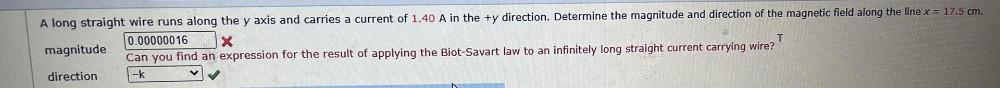Question:

# T A long straight wire runs along the y axis and carries a current of 1.40 A in the +y direction. Determine the magnitude and diT A long straight wire runs along the y axis and carries a current of 1.40 A in the +y direction. Determine the magnitude and direction of the magnetic field along the line x = 17.5 cm. 0.00000016 X magnitude Can you find an expression for the result of applying the Biot-Savart law to an infinitely long straight current carrying wire? direction -K